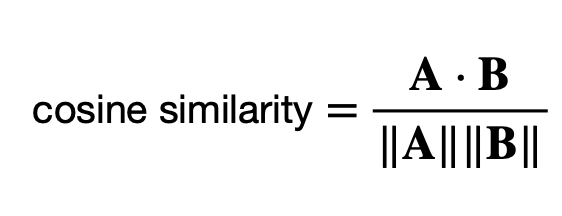## Pages

### Cosine Similarity Computing Example with SpaCy

Cosine similarity is a powerful metric with wide-ranging applications in natural language processing, information retrieval, recommendation systems, and more. It enables us to quantify the similarity or dissimilarity between two non-zero vectors in a multi-dimensional space. In the realm of text data, cosine similarity plays a vital role in measuring the similarity between documents or sentences.

In this blog post, we will explore cosine similarity and its applications using the SpaCy library. We'll delve into the fundamental concept of cosine similarity, and show you how to compute it using Spacy. The tutorial covers:

1. The concept of cosine similarity
2. Installing SpaCy
3. Computing Cosine Similarity with SpaCy
4. Conclusion

Let's get started.

The concept of cosine similarity

Cosine similarity is a metric used to measure how similar two non-zero vectors are in a multi-dimensional space. It's often employed in various fields, including natural language processing, document retrieval, and recommendation systems. Cosine similarity quantifies the cosine of the angle between two vectors, and it ranges from -1 (completely dissimilar) to 1 (completely similar), with 0 indicating no similarity.

Here's how cosine similarity works in the context of text data, which is a common application:

Vector Representation: Documents or sentences are represented as vectors in a multi-dimensional space. Each dimension typically corresponds to a word or a term, and the value of each dimension represents the importance or frequency of that word in the document. One common vectorization technique is TF-IDF (Term Frequency-Inverse Document Frequency).

Cosine of the Angle: The cosine similarity between two vectors is computed by taking the dot product of the vectors and dividing it by the product of their magnitudes (lengths). Mathematically, it's defined as:Where:
A ⋅ B is the dot product of vectors A and B.
||A|| and ||B|| are the magnitudes (lengths) of vectors A and B.

Interpreting the Result: If the vectors are identical (point in the same direction), the cosine similarity is 1, indicating they are perfectly similar. If the vectors are orthogonal (at a 90-degree angle), the cosine similarity is 0, indicating no similarity. If the vectors are diametrically opposed (point in opposite directions), the cosine similarity is -1, indicating they are completely dissimilar.

Installing SpaCy

Before we dive into the code, you'll need to install SpaCy, a popular Python library for natural language processing. You can install it using pip:

pip install spacy

Once installed, you'll need to download a language model for SpaCy. For this example, we'll use the English model, but SpaCy supports multiple languages, and you can choose the one that suits your needs. Download the English model with:

Computing cosine similarity with SpaCy

Now, let's see how to compute cosine similarity with SpaCy. We'll use the en_core_web_sm model for text processing and compute the similarity between an input phrase and a set of standard phrases. We first load the English language model with SpaCy, and then we process the input phrase and standard phrases using the model. We compute cosine similarity using the vectors associated with these processed documents. The result will be the cosine similarity scores for each standard phrase.

` `
import spacy
import numpy as np

# Load the SpaCy English model with word vectors

# Sample phrases
standard_phrases = [
"Code efficiently",
"Debug with patience",
"Think logically",
]

# Define a new input phrase
input_phrase = "Fix all bugs quickly."

# Process the input phrase and compute its vector
input_doc = nlp(input_phrase)
input_vector = input_doc.vector

# Process and compute vectors for standard phrases
standard_vectors = [nlp(phrase).vector for phrase in standard_phrases]

# Calculate cosine similarity between the input vector and standard vectors
cos_sim_scores = [np.dot(input_vector, sv) / (np.linalg.norm(input_vector)
* np.linalg.norm(sv)) for sv in standard_vectors]

# Print the cosine similarity scores for each standard phrase
for ph, score in zip(standard_phrases, cos_sim_scores):
print(f"Similarity between '{input_phrase}' and '{ph}': {score:.4f}")
` `
` `

And result looks as below.

Similarity between 'Fix all bugs quickly.' and 'Code efficiently': 0.5538
Similarity between 'Fix all bugs quickly.' and 'Debug with patience': 0.1817
Similarity between 'Fix all bugs quickly.' and 'Automate task': 0.1789
Similarity between 'Fix all bugs quickly.' and 'Think logically': 0.2591

Conclusion

In this tutorial, we've explored the concept of cosine similarity and learned how to implement it with SpaCy. Cosine similarity is a versatile metric that helps in measuring and understanding similarity relationships in multi-dimensional spaces, making it a valuable asset in applications such as document retrieval, recommendation systems, and text classification. Understanding and effectively implementing cosine similarity can greatly enhance the capabilities of natural language processing and text analysis projects.

References: